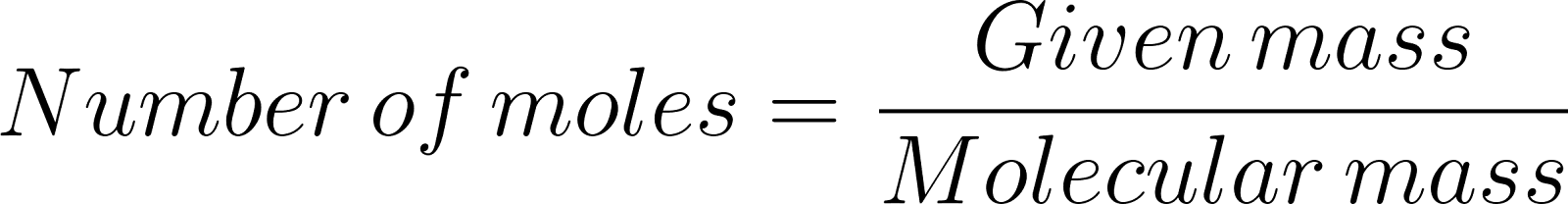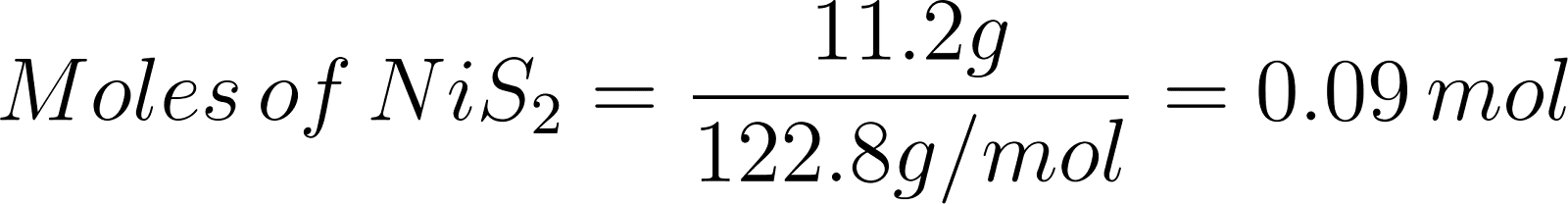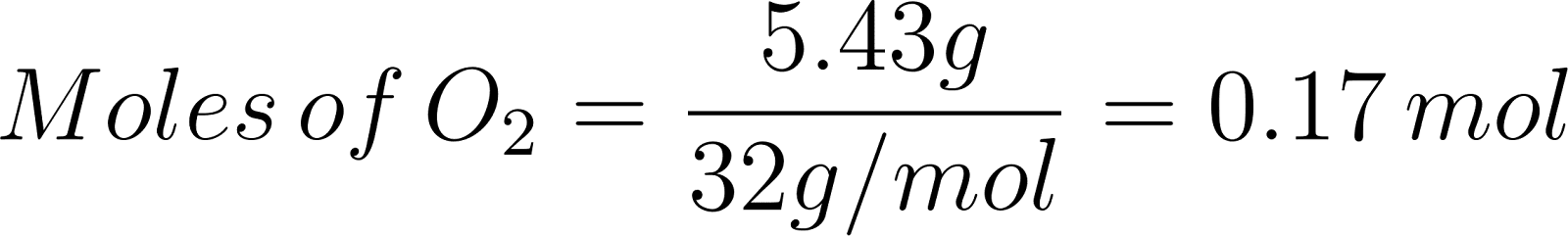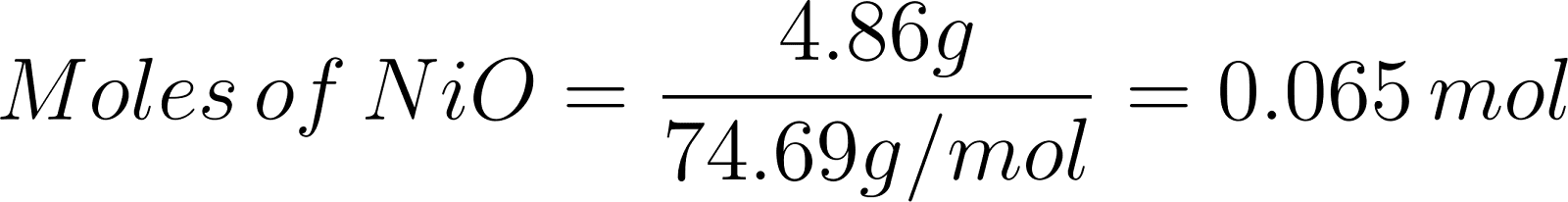×
Get Full Access to Introductory Chemistry - 5 Edition - Chapter 8 - Problem 63p
Get Full Access to Introductory Chemistry - 5 Edition - Chapter 8 - Problem 63p

×

# Consider the reaction between NiS2 and O2:2 NiS2(s) + 5 O2(g) ? 2 NiO(s) + 4 SO2(g)WhenISBN: 9780321910295 34

## Solution for problem 63P Chapter 8

Introductory Chemistry | 5th Edition

• Textbook Solutions
• 2901 Step-by-step solutions solved by professors and subject experts
• Get 24/7 help from StudySoup virtual teaching assistantsIntroductory Chemistry | 5th Edition

4 5 1 289 Reviews
31
3
Problem 63P

PROBLEM 63P

Consider the reaction between NiS2 and O2:

2 NiS2(s) + 5 O2(g) → 2 NiO(s) + 4 SO2(g)

When 11.2 g of NiS2 react with 5.43 g of O2, 4.86 g of NiO are obtained. Determine the limiting reactant, theoretical yield of NiO, and percent yield for the reaction.

Step-by-Step Solution:

Problem 63P

PROBLEM 63P

Consider the reaction between NiS2 and O2:

2 NiS2(s) + 5 O2(g) ? 2 NiO(s) + 4 SO2(g)

When 11.2 g of NiS2 react with 5.43 g of O2, 4.86 g of NiO are obtained. Determine the limiting reactant, theoretical yield of NiO, and percent yield for the reaction.

Step by step solution

Step 1 of 3

The given chemical reaction isa s follows.Let’s calculate the moles of reactants and products.

Moles can be calculated by the following formula.Step 2 of 3

Step 3 of 3

##### ISBN: 9780321910295

The full step-by-step solution to problem: 63P from chapter: 8 was answered by , our top Chemistry solution expert on 05/06/17, 06:45PM. The answer to “Consider the reaction between NiS2 and O2:2 NiS2(s) + 5 O2(g) ? 2 NiO(s) + 4 SO2(g)When 11.2 g of NiS2 react with 5.43 g of O2, 4.86 g of NiO are obtained. Determine the limiting reactant, theoretical yield of NiO, and percent yield for the reaction.” is broken down into a number of easy to follow steps, and 47 words. This full solution covers the following key subjects: nio, nis, reaction, Yield, obtained. This expansive textbook survival guide covers 19 chapters, and 2046 solutions. This textbook survival guide was created for the textbook: Introductory Chemistry, edition: 5. Introductory Chemistry was written by and is associated to the ISBN: 9780321910295. Since the solution to 63P from 8 chapter was answered, more than 713 students have viewed the full step-by-step answer.

Unlock Textbook Solution